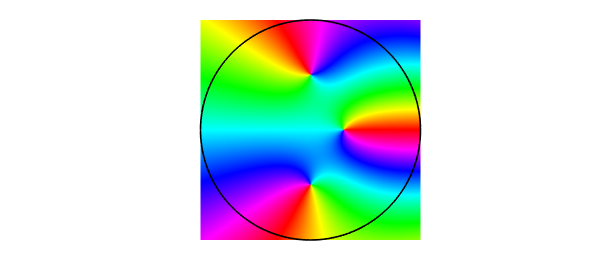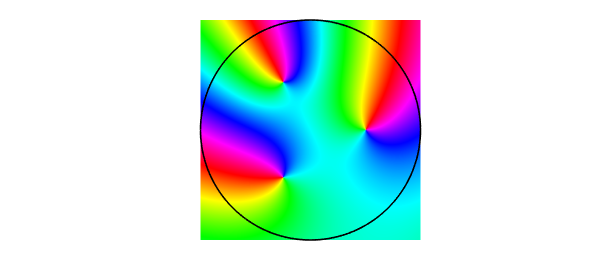function ComplexRoots

Poles and zeros of complex functions can be located by the evaluation of contour integrals, as mentioned in Chapter 5 of the Chebfun Guide. For example, suppose we have a function like this one with a single root $s_1$ in the unit disk:

ff = @(z) (z-0.5i).*exp(z);

We can find the root as the value of a contour integral around the unit circle: $$s = \frac{1}{2i\pi} \int z \frac{f'(z)}{f(z)} dz.$$

Since Chebfun works with real independent variables, we parametrize the unit circle by a real variable $t$ on $[-1,1]$:

z = chebfun('exp(1i*pi*t)');

which gives us $$s_1 = \frac{1}{2i\pi} \int z \frac{(df/dt)(dt/dz)}{f} \frac{dz}{dt} dt$$ $$\phantom{s_1} = \frac{1}{2i\pi} \int z \frac{(df/dt)}{f} dt.$$

So here is the Chebfun evaluation:

f = ff(z);
s1 = sum(z.*diff(f)./f)/(2i*pi)
s1 =
-0.000000000000003 + 0.500000000000002i


There is nothing in this computation that depends on the use of the unit disk. Other contours are equally tracatable in Chebfun, as illustrated in the Example complex/KeyholeContour and in Chapter 5 of the Chebfun Guide.

This method of finding a single root goes back at least to McCune in 1966 . In practice we would often want to be able to find multiple roots, and a generalized algorithm for this case was published by Delves and Lyness in 1967 , with mathematical origins as far back as Jackson in 1917 . For a discussion of all kinds of related algorithms, see . The idea here is that if $f$ has more than one root in the unit disk, then the value $s$ above comes out as the sum of all these roots. Similarly

$$s_2 = \frac{1}{2i\pi} \int z^2 \frac{f'(z)}{f(z)} dz$$

is the sum of the squares of the roots, the analogous formula for $s_3$ with a factor $z^3$ gives the sum of the cubes, and so on. And a count of the number of roots is given by

$$s_0 = \frac{1}{2i\pi} \int \frac{f'(z)}{f(z)} dz$$

(this is basically the argument principle). So for example we can count the number of roots of $\cosh(\pi z)$ in the unit disk like this:

ff = @(z) cosh(pi*z);
f = ff(z);
s0 = sum(diff(f)./f)/(2i*pi)
s0 =
2.000000000000000 - 0.000000000000000i


Here are the sum of the roots and the sum of their squares:

s1 = sum(z.*diff(f)./f)/(2i*pi)
s2 = sum(z.^2.*diff(f)./f)/(2i*pi)
s1 =
4.330727237839987e-20 - 1.370796878478212e-17i
s2 =
-0.499999999999999 + 0.000000000000000i


corresponding to roots at $\pm 0.5i$. We can find these numbers systematically by noting that the monic polynomial $p(z)$ with these roots has coefficients $c_0 = (s_1^2-s_2)/2$, $c_1=-s_1$, $c_2=1$. So here is a calculation of the two roots in the unit disk of $\cosh(\pi z)$:

p = [1 -s1 (s1^2-s2)/2];
roots(p)
ans =
-0.000000000000000 - 0.499999999999999i
0.000000000000000 + 0.499999999999999i


Generalization to higher numbers of roots can be done via Newton's identities. We don't pursue the general case here but instead write a code that finds three roots of an analytic function in the unit disk:

function r = roots3(ff)    % find 3 roots of ff in unit disk
z = chebfun('exp(1i*pi*t)');
f = ff(z);
s0 = sum(diff(f)./f)/(2i*pi);
s1 = sum(z.*diff(f)./f)/(2i*pi);
s2 = sum(z.^2.*diff(f)./f)/(2i*pi);
s3 = sum(z.^3.*diff(f)./f)/(2i*pi);
p = [1 -s1 (s1^2-s2)/2 -(s1^3-3*s1*s2+2*s3)/6];
r = roots(p);
end

Here is an example, this time with a phase portrait to visually verify the roots:

ff = @(z) cosh(exp(z)).*(z-.3).*(1+4*z.^2);
roots3(ff)
plot(chebfun2(ff)), hold on
plot(z, 'k-'), axis equal
ans =
-0.000000000000000 + 0.500000000000000i
0.000000000000000 - 0.499999999999999i
0.299999999999999 - 0.000000000000000iHere is another:

ff = @(z) (z.^3-1/8).*exp((-1-2i)*z);
roots3(ff)
plot(chebfun2(ff)), hold on
plot(z, 'k-'), axis equal
ans =
-0.250000000000002 + 0.433012701892223i
0.500000000000001 + 0.000000000000005i
-0.249999999999994 - 0.433012701892219iend
1. A. P. Austin, P. Kravanja and L. N. Trefethen, Numerical algorithms based on analytic function values at roots of unity, SIAM Journal on Numerical Analysis, to appear.

2. L. M. Delves and J. N. Lyness, A numerical method for lcoating the zeros of an analytic function, Mathematics of Computation 21 (1967), 543-560.

3. D. Jackson, Roots and singular points of analytic functions, Annals of Matheamtics 19 (1917), 142-151.

4. J. E. McCune, Exact inversion of dispersion relations, Physics of Fluids 9 (1966), 2082-2084.